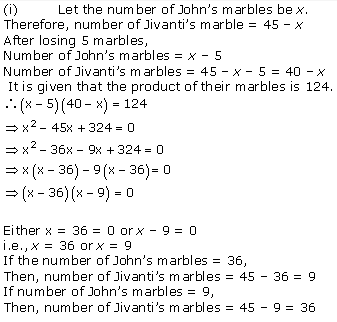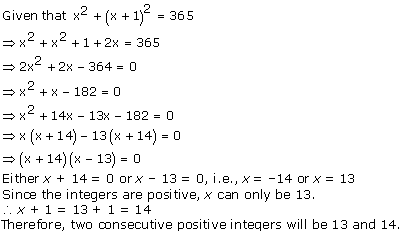# NCERT Solutions for Class 10 Maths Chapter 4 Quadratic Equations Ex 4.2

NCERT Solutions for Class 10 Maths Chapter 4 Quadratic Equations Ex 4.2 are part of . Here are we have given Chapter 4 Quadratic Equations Class 10 NCERT Solutions Ex 4.2.

 Board CBSE Textbook NCERT Class Class 10 Subject Maths Chapter Chapter 4 Chapter Name Quadratic Equations Exercise Ex 4.2 Number of Questions Solved 6 Category NCERT Solutions

## NCERT Solutions for Class 10 Maths Chapter 4 Quadratic Equations Ex 4.2

Page No: 76

Question 1. Find the roots of the following quadratic equations by factorisation:
(i) x2 – 3x – 10 = 0
(ii) 2x2 + x – 6 = 0
(iii) √2 x2 + 7x + 5√2 = 0
(iv) 2x2x + 1/8 = 0
(v) 100x2 – 20x + 1 = 0

Solution :Concept Insight: Two solve a quadratic equation by factorisation method two key results are used (i) To split the middle term find the numbers a and b such that their sum is equal to the coeffident of x and product is equal to the product of coefficients of x2 and constant term. (ii) Product of num bers zero means either or both are zero.

Question 2.
(i) John and Jivanti together have 45 marbles. Both of them lost 5 marbles each, and the product of the number of marbles they now have is 124. Find out how many marbles they had to start with.

Solution :(ii) A cottage industry produces a certain number of toys in a day. The cost of production of each toy (in rupees) was found to be 55 minus the number of toys produced in a day. On a particular day, the total cost of production was Rs 750. Find out the number of toys produced on that day.

Solution :Concept Insight: Read the question carefully and choose the variable to represent what needs to be found. Only one variable must be there in the final equation.

Question 3. Find two numbers whose sum is 27 and product is 182.

Solution :Question 4. Find two consecutive positive integers, sum of whose squares is 365.

Solution :Question 5. The altitude of a right triangle is 7 cm less than its base. If the hypotenuse is 13 cm, find the other two sides.

Solution :Question 6. A cottage industry produces a certain number of pottery articles in a day. It was observed on a particular day that the cost of production of each article (in rupees) was 3 more than twice the number of articles produced on that day. If the total cost of production on that day was Rs 90, find the number of articles produced and the cost of each article.

Solution :We hope the NCERT Solutions for Class 10 Maths Chapter 4 Quadratic Equations Ex 4.2 help you. If you have any query regarding NCERT Solutions for Class 10 Maths Chapter 4 Quadratic Equations Ex 4.2, drop a comment below and we will get back to you at the earliest.